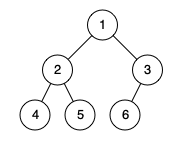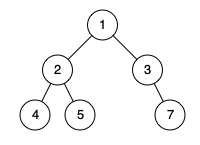958. Check Completeness of a Binary Tree

Given the `root` of a binary tree, determine if it is a complete binary tree.

In a complete binary tree, every level, except possibly the last, is completely filled, and all nodes in the last level are as far left as possible. It can have between `1` and `2h` nodes inclusive at the last level `h`.

Example 1:```Input: root = [1,2,3,4,5,6]
Output: true
Explanation: Every level before the last is full (ie. levels with node-values {1} and {2, 3}), and all nodes in the last level ({4, 5, 6}) are as far left as possible.
```

Example 2:```Input: root = [1,2,3,4,5,null,7]
Output: false
Explanation: The node with value 7 isn't as far left as possible.
```

Constraints:

• The number of nodes in the tree is in the range `[1, 100]`.
• `1 <= Node.val <= 1000`

958. Check Completeness of a Binary Tree
``````struct Solution;
use rustgym_util::*;
use std::collections::HashSet;

trait Postorder {
fn postorder(&self, id: u32, nodes: &mut HashSet<u32>) -> usize;
}

fn postorder(&self, id: u32, nodes: &mut HashSet<u32>) -> usize {
if let Some(node) = self {
let node = node.borrow();
let left = node.left.postorder(id << 1, nodes);
let right = node.right.postorder((id << 1) | 1, nodes);
nodes.insert(id);
left + right + 1
} else {
0
}
}
}

impl Solution {
fn is_complete_tree(root: TreeLink) -> bool {
let mut nodes: HashSet<u32> = HashSet::new();
let count = root.postorder(1, &mut nodes);
nodes.len() == count && nodes.into_iter().all(|x| x <= count as u32)
}
}

#[test]
fn test() {
let root = tree!(1, tree!(2, tree!(4), tree!(5)), tree!(3, tree!(6), None));
let res = true;
assert_eq!(Solution::is_complete_tree(root), res);
let root = tree!(1, tree!(2, tree!(4), tree!(5)), tree!(3, None, tree!(7)));
let res = false;
assert_eq!(Solution::is_complete_tree(root), res);
}
``````